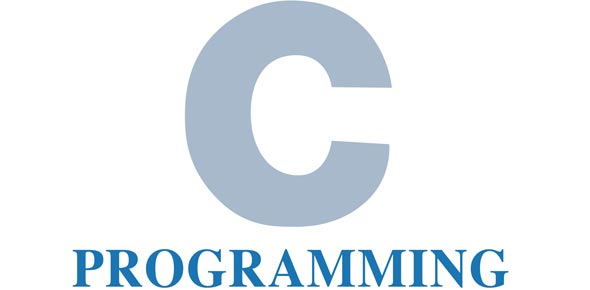# Is Ch 1-3 Test C++ Your Forte? Find Out With This Quiz!

26 Questions | Total Attempts: 63SettingsC++ language was developed in 1979 and it is a sophisticated general purpose programming language based on C. If you are a starter at this language, then the quiz below is perfect for you, if you consider chapter one to three your forte. Take up the quiz and prove yourself!

• 1.
1. Change the following for loop into a while loop
for( i=0;i < 10; i+=3) {     sum += i; }
• 2.
Explain the difference between a do-while loop and a while loop.
• 3.
Write a complete program that will convert from inches to centimeters. Use the formula     cent = inches * 2.54
• 4.
1. Write a program to add and average test scores for this test. Use a sentinel controlled loop to do this. Do not worry about comments and the intial comment section. This is a complete program.
• 5.
1. Write a switch statement to determine the GPA of a student given the letter grade. Use the following table as a guide.  Double gpa=0,class=0; Letter GPA      A 4.0 B 3.0 C 2.0 D 1.0 F 0.0
• 6.
1. Write the program again using if-else statements. Determine the GPA of a student given the letter grade. Use the following table as a guide.   Double gpa=0,class=0; Letter GPA      A 4.0 B 3.0 C 2.0 D 1.0 F 0.0
• 7.
If the following are valid identifiers, check valid, otherwise check invalid, return
• A.

Valid

• B.

Invalid

• 8.
STUDENTS
• A.

Valid

• B.

Invalid

• 9.
• A.

Valid

• B.

Invalid

• 10.
• A.

Valid

• B.

Invalid

• 11.
2file
• A.

Valid

• B.

Invalid

• 12.
Cpu
• A.

Valid

• B.

Invalid

• 13.
Counter value
• A.

Valid

• B.

Invalid

• 14.
Sprint.L.L.C
• A.

Valid

• B.

Invalid

• 15.
Counter4Data
• A.

Valid

• B.

Invalid

• 16.
Write only the code necessary to declare a variable that would hold the cost of an automobile.  Initialize it with a value of 0;
• A.

Int cost = 0;

• B.

Double cost = 0;

• C.

Cost = 0;

• D.

Double = 0;

• 17.
Evaluate the following numerical expression. int number; int x = 9, y = 5; float ave = 13.5 number = ( x + y / 3 * 2 ) + ave / x -1 * ave;
• 18.
Evaluate the following logical expressions as true or false: int x=0, y=1; bool value=false;   (these values are the same for each expression).         (x=0 && y < 5/3 + 3 || value == 0/y)
• A.

True

• B.

False

• 19.
Evaluate the following logical expressions as true or false: int x=0, y=1; bool value=false;   (these values are the same for each expression).     (x + y || value && x || !value)
• A.

True

• B.

False

• 20.
Assume that for each operation, a is 5, b is 14, and c is 8. a, b and c are all integers. a % b
• 21.
Assume that for each operation, a is 5, b is 14, and c is 8. a, b and c are all integers. a / b
• 22.
Assume that for each operation, a is 5, b is 14, and c is 8. a, b and c are all integers. float(a) / c
• 23.
Assume that for each operation, a is 5, b is 14, and c is 8. a, b and c are all integers. float(a / b)
• 24.
1. What is the output of the following code fragment?
int i=20;   do {     cout << i << i+1 << endl; }while(i > 10);
• A.

20 21

• B.

10 20 21

• C.

20 21 repeating

• 25.
What is wrong with the following code that will assign my first initial to a variable? char initial; initial=M;
• A.

The m needs to have ‘’ around it "M".

• B.

The m needs to have ‘’ around it ‘M’.

• C.

It is fine.

Related TopicsBack to top